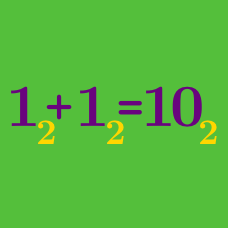Number Theory

# Number Bases: Level 3 Challenges

There is a number $a$, which can be written as $\overline{xyz}$ in base 9 and $\overline{zyx}$ in base 6, for some positive integer $x,y,z$. Find $x+y+z$.

Details and Assumption

$\overline{xyz}$ represents reading the digits together, instead of multiplying them out. For example, $\overline{xyz}_9 = 81 x + 9y + z$ and $\overline{zyx}_6 = 36 z + 6y + x$.

Find the smallest natural number greater than 3 which has the unit digit of 3 when expressed in base-4, base-5, base-6, base-7, and base-8.

$N$ is an integer whose representation in base $b$ is 777. Find the smallest positive integer $b$ for which $N$ is the fourth power of an integer.

$(2^3)! = 40320_{10} = 1001\:1101\:1000\:0000_2.$

The factorial shown above has seven trailing zeros.

How many trailing zeroes does the number $(2^{16})!$ have in binary notation?

$1,3,4,9,10,12,13,\ldots$

The above sequence consists of the powers of $3$ or the sum of distinct powers of $3$, arranged in increasing order.

Find the $100^\text{th}$ term of the sequence.

×